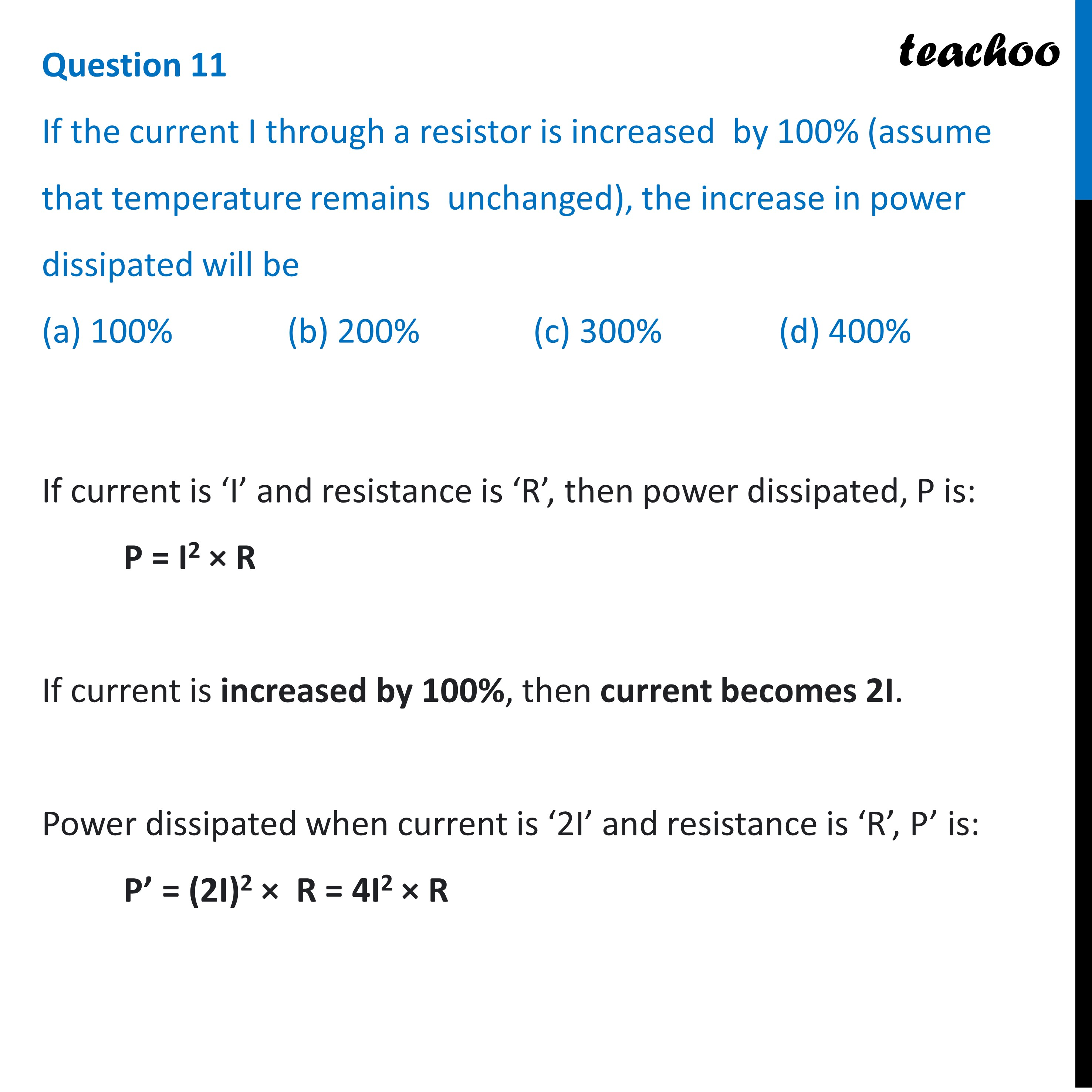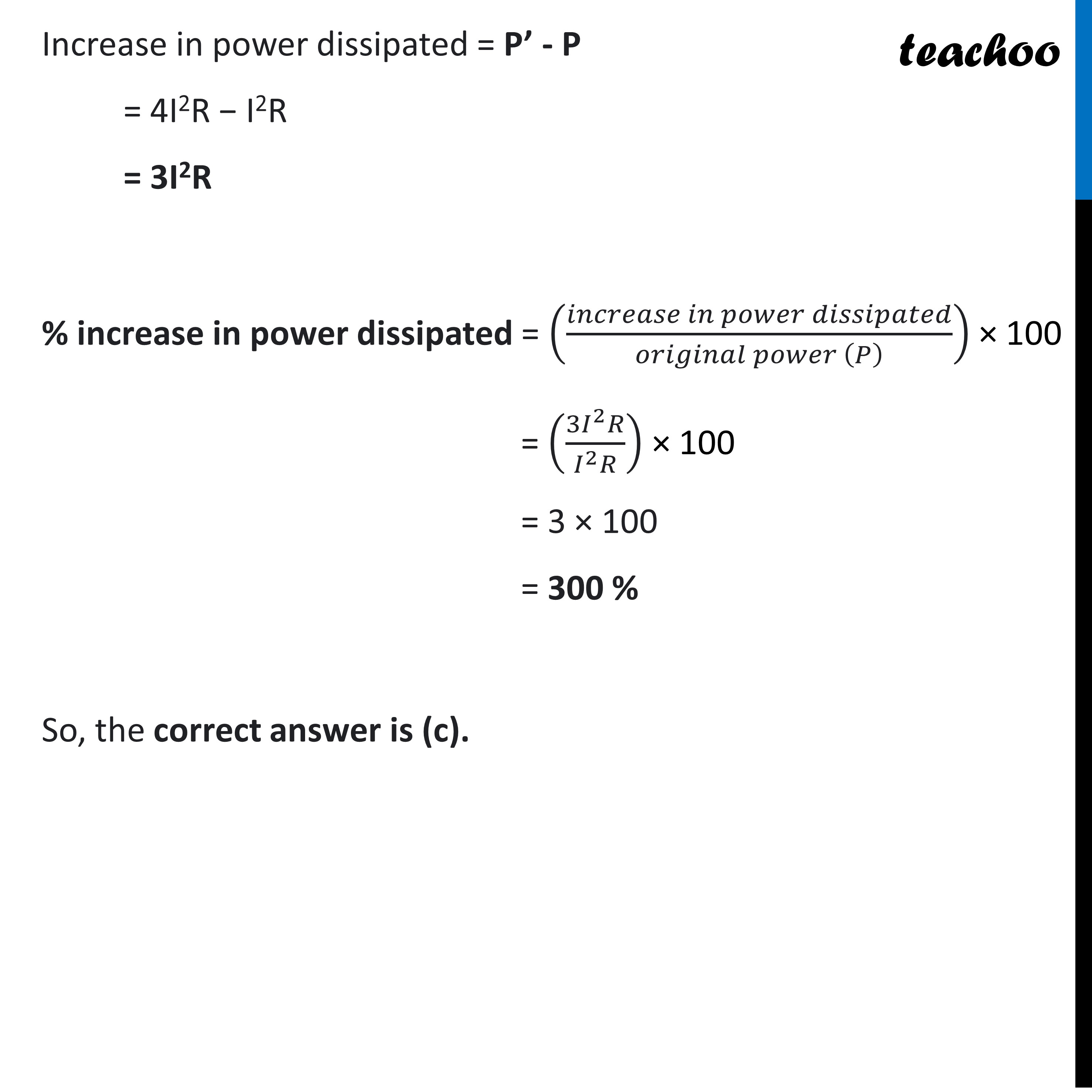MCQs from (Past Year Papers & NCERT Exemplar)

Class 10
Chapter 12 Class 10 - Electricity

## (d) 400%If current is ‘I’ and resistance is ‘R’, then power dissipated, P is:

P = I 2 * R

If current is increased by 100% , then current becomes 2I .

Power dissipated when current is ‘2I’ and resistance is ‘R’, P’ is:

P’ = (2I) 2 * R = 4I 2 * R

Increase in power dissipated = P’ - P

= 4I 2 R - I 2 R

= 3I 2 R

% increase in power dissipated = (increase in power dissipated / original power(P) ) * 100

= (3I 2 R / I 2 R ) * 100

= 3 * 100

= 300 %

So, the correct answer is (c).

Learn in your speed, with individual attention - Teachoo Maths 1-on-1 Class

### Transcript

Question 11 If the current I through a resistor is increased by 100% (assume that temperature remains unchanged), the increase in power dissipated will be (a) 100% (b) 200% (c) 300% (d) 400%If current is ‘I’ and resistance is ‘R’, then power dissipated, P is: P = I2 × R If current is increased by 100%, then current becomes 2I. Power dissipated when current is ‘2I’ and resistance is ‘R’, P’ is: P’ = (2I)2 × R = 4I2 × R Increase in power dissipated = P’ - P = 4I2R − I2R = 3I2R % increase in power dissipated = ((𝑖𝑛𝑐𝑟𝑒𝑎𝑠𝑒 𝑖𝑛 𝑝𝑜𝑤𝑒𝑟 𝑑𝑖𝑠𝑠𝑖𝑝𝑎𝑡𝑒𝑑)/(𝑜𝑟𝑖𝑔𝑖𝑛𝑎𝑙 𝑝𝑜𝑤𝑒𝑟 (𝑃) )) × 100 = ((3𝐼^2 𝑅)/(𝐼^2 𝑅)) × 100 = 3 × 100 = 300 % So, the correct answer is (c).